x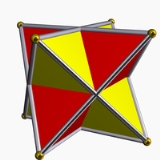Polyhedral compoundEncyclopedia
A polyhedral compound is a polyhedron
Polyhedron
In elementary geometry a polyhedron is a geometric solid in three dimensions with flat faces and straight edges...

that is itself composed of several other polyhedra sharing a common centre. They are the three-dimensional analogs of polygonal compounds such as the hexagram.

Neighbouring vertices of a compound can be connected to form a convex polyhedron called the convex hull
Convex hull
In mathematics, the convex hull or convex envelope for a set of points X in a real vector space V is the minimal convex set containing X....

. The compound is a facetting
Facetting
In geometry, facetting is the process of removing parts of a polygon, polyhedron or polytope, without creating any new vertices.Facetting is the reciprocal or dual process to stellation...

of the convex hull.

Another convex polyhedron is formed by the small central space common to all members of the compound. This polyhedron can be considered the core for a set of stellation
Stellation
Stellation is a process of constructing new polygons , new polyhedra in three dimensions, or, in general, new polytopes in n dimensions. The process consists of extending elements such as edges or face planes, usually in a symmetrical way, until they meet each other again...

s including this compound. (See List of Wenninger polyhedron models for these compounds and more stellations.)

## Regular compounds

A regular polyhedron compound can be defined as a compound which, like a regular polyhedron, is vertex-transitive
Vertex-transitive
In geometry, a polytope is isogonal or vertex-transitive if, loosely speaking, all its vertices are the same...

, edge-transitive, and face-transitive. With this definition there are 5 regular compounds.
Components Picture Convex hull
Convex hull
In mathematics, the convex hull or convex envelope for a set of points X in a real vector space V is the minimal convex set containing X....

Core Symmetry
Symmetry group
The symmetry group of an object is the group of all isometries under which it is invariant with composition as the operation...

Dual
Compound of two tetrahedra, or stella octangula
Stella octangula
The stellated octahedron, or stella octangula, is the only stellation of the octahedron. It was named by Johannes Kepler in 1609, though it was known to earlier geometers...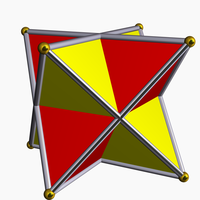Cube  Octahedron
Octahedron
In geometry, an octahedron is a polyhedron with eight faces. A regular octahedron is a Platonic solid composed of eight equilateral triangles, four of which meet at each vertex....

Oh Self-dual
Compound of five tetrahedra
Compound of five tetrahedra
This compound polyhedron is also a stellation of the regular icosahedron. It was first described by Edmund Hess in 1876.-As a compound:It can be constructed by arranging five tetrahedra in rotational icosahedral symmetry , as colored in the upper right model...

, or chiro-icosahedron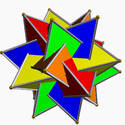Dodecahedron  Icosahedron
Icosahedron
In geometry, an icosahedron is a regular polyhedron with 20 identical equilateral triangular faces, 30 edges and 12 vertices. It is one of the five Platonic solids....

I enantiomorph
Chirality (mathematics)
In geometry, a figure is chiral if it is not identical to its mirror image, or, more precisely, if it cannot be mapped to its mirror image by rotations and translations alone. For example, a right shoe is different from a left shoe, and clockwise is different from counterclockwise.A chiral object...

, or chiral
Chirality (mathematics)
In geometry, a figure is chiral if it is not identical to its mirror image, or, more precisely, if it cannot be mapped to its mirror image by rotations and translations alone. For example, a right shoe is different from a left shoe, and clockwise is different from counterclockwise.A chiral object...

twin
Compound of ten tetrahedra
Compound of ten tetrahedra
This polyhedron can be seen as either a polyhedral stellation or a compound. This compound was first described by Edmund Hess in 1876.- As a compound :It can also be seen as the compound of ten tetrahedra with full icosahedral symmetry...

, compound of two chiro-icoshedra, or icosiicosahedron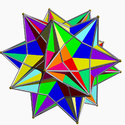Dodecahedron  Icosahedron
Icosahedron
In geometry, an icosahedron is a regular polyhedron with 20 identical equilateral triangular faces, 30 edges and 12 vertices. It is one of the five Platonic solids....

Ih Self-dual
Compound of five cubes
Compound of five cubes
This polyhedral compound is a symmetric arrangement of five cubes. This compound was first described by Edmund Hess in 1876.It is one of five regular compounds, and dual to the compound of five octahedra....

, or rhombihedron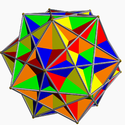Dodecahedron Rhombic triacontahedron
Rhombic triacontahedron
In geometry, the rhombic triacontahedron is a convex polyhedron with 30 rhombic faces. It is an Archimedean dual solid, or a Catalan solid. It is the polyhedral dual of the icosidodecahedron, and it is a zonohedron....

Ih Compound of five octahedra
Compound of five octahedra
This polyhedron can be seen as either a polyhedral stellation or a compound. This compound was first described by Edmund Hess in 1876.- As a stellation :It is the second stellation of the icosahedron, and given as Wenninger model index 23....

Compound of five octahedra
Compound of five octahedra
This polyhedron can be seen as either a polyhedral stellation or a compound. This compound was first described by Edmund Hess in 1876.- As a stellation :It is the second stellation of the icosahedron, and given as Wenninger model index 23....

, or small icosiicosahedron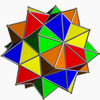Icosidodecahedron
Icosidodecahedron
In geometry, an icosidodecahedron is a polyhedron with twenty triangular faces and twelve pentagonal faces. An icosidodecahedron has 30 identical vertices, with two triangles and two pentagons meeting at each, and 60 identical edges, each separating a triangle from a pentagon...

Icosahedron
Icosahedron
In geometry, an icosahedron is a regular polyhedron with 20 identical equilateral triangular faces, 30 edges and 12 vertices. It is one of the five Platonic solids....

Ih Compound of five cubes
Compound of five cubes
This polyhedral compound is a symmetric arrangement of five cubes. This compound was first described by Edmund Hess in 1876.It is one of five regular compounds, and dual to the compound of five octahedra....

Best known is the compound of two tetrahedra
Tetrahedron
In geometry, a tetrahedron is a polyhedron composed of four triangular faces, three of which meet at each vertex. A regular tetrahedron is one in which the four triangles are regular, or "equilateral", and is one of the Platonic solids...

, often called the stella octangula
Stella octangula
The stellated octahedron, or stella octangula, is the only stellation of the octahedron. It was named by Johannes Kepler in 1609, though it was known to earlier geometers...

, a name given to it by Kepler
Johannes Kepler
Johannes Kepler was a German mathematician, astronomer and astrologer. A key figure in the 17th century scientific revolution, he is best known for his eponymous laws of planetary motion, codified by later astronomers, based on his works Astronomia nova, Harmonices Mundi, and Epitome of Copernican...

. The vertices of the two tetrahedra define a cube and the intersection of the two an octahedron
Octahedron
In geometry, an octahedron is a polyhedron with eight faces. A regular octahedron is a Platonic solid composed of eight equilateral triangles, four of which meet at each vertex....

, which shares the same face-planes as the compound. Thus it is a stellation
Stellation
Stellation is a process of constructing new polygons , new polyhedra in three dimensions, or, in general, new polytopes in n dimensions. The process consists of extending elements such as edges or face planes, usually in a symmetrical way, until they meet each other again...

of the octahedron, and in fact, the only finite stellation thereof.

The stella octangula can also be regarded as a dual-regular compound.

The compound of five tetrahedra
Compound of five tetrahedra
This compound polyhedron is also a stellation of the regular icosahedron. It was first described by Edmund Hess in 1876.-As a compound:It can be constructed by arranging five tetrahedra in rotational icosahedral symmetry , as colored in the upper right model...

comes in two enantiomorph
Chirality (mathematics)
In geometry, a figure is chiral if it is not identical to its mirror image, or, more precisely, if it cannot be mapped to its mirror image by rotations and translations alone. For example, a right shoe is different from a left shoe, and clockwise is different from counterclockwise.A chiral object...

ic versions, which together make up the compound of 10 tetrahedra. Each of the tetrahedral compounds is self-dual, and the compound of 5 cubes is dual to the compound of 5 octahedra.

## Dual-regular compounds

A dual-regular compound is composed of a regular polyhedron (one of the Platonic solid
Platonic solid
In geometry, a Platonic solid is a convex polyhedron that is regular, in the sense of a regular polygon. Specifically, the faces of a Platonic solid are congruent regular polygons, with the same number of faces meeting at each vertex; thus, all its edges are congruent, as are its vertices and...

s or Kepler-Poinsot polyhedra) and its regular dual, arranged reciprocally about a common intersphere or midsphere, such that the edge of one polyhedron intersects the dual edge of the dual polyhedron. There are five such compounds.
Components Picture Convex hull
Convex hull
In mathematics, the convex hull or convex envelope for a set of points X in a real vector space V is the minimal convex set containing X....

Core Symmetry
Symmetry group
The symmetry group of an object is the group of all isometries under which it is invariant with composition as the operation...

Compound of two tetrahedra, or Stella octangulaCube
Cube
In geometry, a cube is a three-dimensional solid object bounded by six square faces, facets or sides, with three meeting at each vertex. The cube can also be called a regular hexahedron and is one of the five Platonic solids. It is a special kind of square prism, of rectangular parallelepiped and...

Octahedron
Octahedron
In geometry, an octahedron is a polyhedron with eight faces. A regular octahedron is a Platonic solid composed of eight equilateral triangles, four of which meet at each vertex....

Oh
Compound of cube and octahedron
Compound of cube and octahedron
This polyhedron can be seen as either a polyhedral stellation or a compound.- As a compound :It can be seen as the compound of an octahedron and a cube...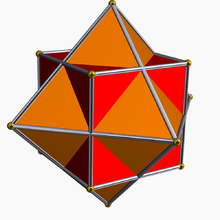Rhombic dodecahedron
Rhombic dodecahedron
In geometry, the rhombic dodecahedron is a convex polyhedron with 12 rhombic faces. It is an Archimedean dual solid, or a Catalan solid. Its dual is the cuboctahedron.-Properties:...

Cuboctahedron
Cuboctahedron
In geometry, a cuboctahedron is a polyhedron with eight triangular faces and six square faces. A cuboctahedron has 12 identical vertices, with two triangles and two squares meeting at each, and 24 identical edges, each separating a triangle from a square. As such it is a quasiregular polyhedron,...

Oh
Compound of dodecahedron and icosahedron
Compound of dodecahedron and icosahedron
In geometry, this polyhedron can be seen as either a polyhedral stellation or a compound.- As a compound :It can be seen as the compound of an icosahedron and dodecahedron...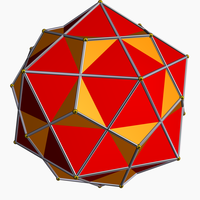Rhombic triacontahedron
Rhombic triacontahedron
In geometry, the rhombic triacontahedron is a convex polyhedron with 30 rhombic faces. It is an Archimedean dual solid, or a Catalan solid. It is the polyhedral dual of the icosidodecahedron, and it is a zonohedron....

Icosidodecahedron
Icosidodecahedron
In geometry, an icosidodecahedron is a polyhedron with twenty triangular faces and twelve pentagonal faces. An icosidodecahedron has 30 identical vertices, with two triangles and two pentagons meeting at each, and 60 identical edges, each separating a triangle from a pentagon...

Ih
Compound of great icosahedron and great stellated dodecahedron
Compound of great icosahedron and great stellated dodecahedron
This polyhedron can be seen as either a polyhedral stellation or a compound.- As a compound :It can be seen as a polyhedron compound of a great icosahedron and great stellated dodecahedron...

Dodecahedron Icosahedron
Icosahedron
In geometry, an icosahedron is a regular polyhedron with 20 identical equilateral triangular faces, 30 edges and 12 vertices. It is one of the five Platonic solids....

Ih
Compound of small stellated dodecahedron and great dodecahedron
Compound of small stellated dodecahedron and great dodecahedron
The compound of small stellated dodecahedron and great dodecahedron is a polyhedron compound where the great dodecahedron is interior to its dual, the small stellated dodecahedron.-See also:*Great dodecahedron*Small stellated dodecahedron...

Icosahedron
Icosahedron
In geometry, an icosahedron is a regular polyhedron with 20 identical equilateral triangular faces, 30 edges and 12 vertices. It is one of the five Platonic solids....

Dodecahedron Ih

The dual-regular compound of a tetrahedron with its dual polyhedron
Dual polyhedron
In geometry, polyhedra are associated into pairs called duals, where the vertices of one correspond to the faces of the other. The dual of the dual is the original polyhedron. The dual of a polyhedron with equivalent vertices is one with equivalent faces, and of one with equivalent edges is another...

is also the regular Stella octangula
Stella octangula
The stellated octahedron, or stella octangula, is the only stellation of the octahedron. It was named by Johannes Kepler in 1609, though it was known to earlier geometers...

, since the tetrahedron is self-dual.

The cube-octahedron and dodecahedron-icosahedron dual-regular compounds are the first stellations of the cuboctahedron
Cuboctahedron
In geometry, a cuboctahedron is a polyhedron with eight triangular faces and six square faces. A cuboctahedron has 12 identical vertices, with two triangles and two squares meeting at each, and 24 identical edges, each separating a triangle from a square. As such it is a quasiregular polyhedron,...

and icosidodecahedron
Icosidodecahedron
In geometry, an icosidodecahedron is a polyhedron with twenty triangular faces and twelve pentagonal faces. An icosidodecahedron has 30 identical vertices, with two triangles and two pentagons meeting at each, and 60 identical edges, each separating a triangle from a pentagon...

, respectively.

The compound of the small stellated dodecahedron and great dodecahedron looks outwardly the same as the small stellated dodecahedron, because the great dodecahedron is completely contained inside. For this reason, the image shown above shows the small stellated dodecahedron in wireframe.

## Uniform compounds

In 1976 John Skilling published Uniform Compounds of Uniform Polyhedra which enumerated 75 compounds (including 6 as infinite prismatic
Prism (geometry)
In geometry, a prism is a polyhedron with an n-sided polygonal base, a translated copy , and n other faces joining corresponding sides of the two bases. All cross-sections parallel to the base faces are the same. Prisms are named for their base, so a prism with a pentagonal base is called a...

sets of compounds, #20-#25) made from uniform polyhedra with rotational symmetry. (Every vertex is vertex-transitive
Vertex-transitive
In geometry, a polytope is isogonal or vertex-transitive if, loosely speaking, all its vertices are the same...

and every vertex is transitive with every other vertex.) This list includes the five regular compounds above. http://www.interocitors.com/polyhedra/UCs/UniformCompounds.html

Here is a pictorial table of the 75 uniform compounds as listed by Skilling. Most are singularly colored by each polyhedron element. Some as chiral pairs are colored by symmetry of the faces within each polyhedron.
• 1-19: Miscellaneous (4,5,6,9,17 are the 5 regular compounds)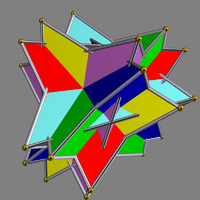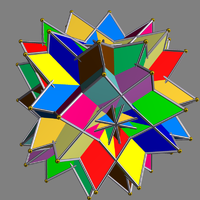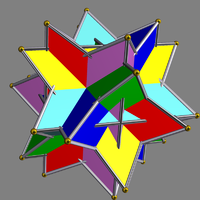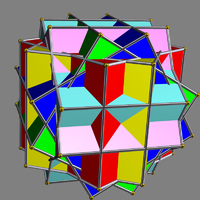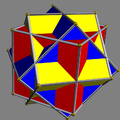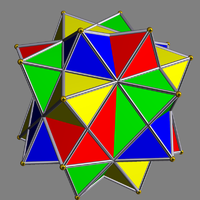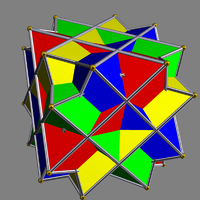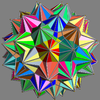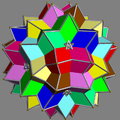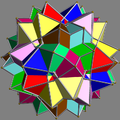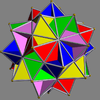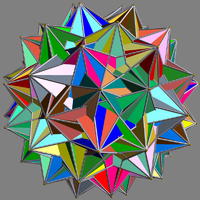• 20-25: Prism symmetry embedded in prism symmetry
Dihedral symmetry in three dimensions
This article deals with three infinite sequences of point groups in three dimensions which have a symmetry group that as abstract group is a dihedral group Dihn .See also point groups in two dimensions.Chiral:...

,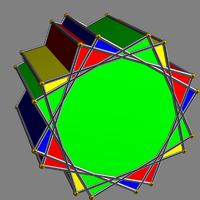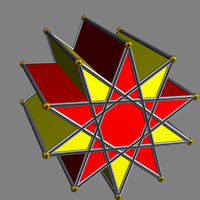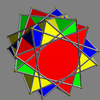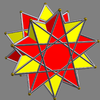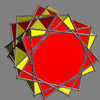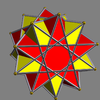• 26-45: Prism symmetry embedded in octahedral
Octahedral symmetry
150px|thumb|right|The [[cube]] is the most common shape with octahedral symmetryA regular octahedron has 24 rotational symmetries, and a symmetry order of 48 including transformations that combine a reflection and a rotation...

or icosahedral symmetry
Icosahedral symmetry
A regular icosahedron has 60 rotational symmetries, and a symmetry order of 120 including transformations that combine a reflection and a rotation...

,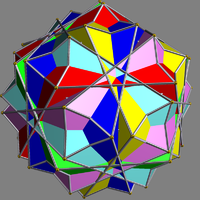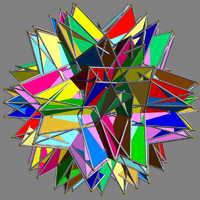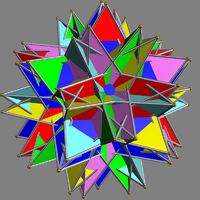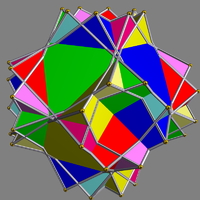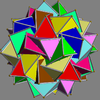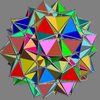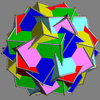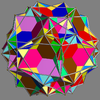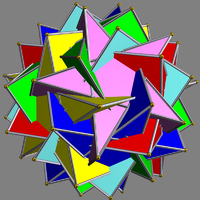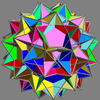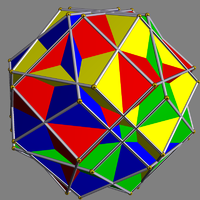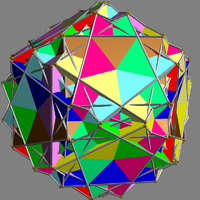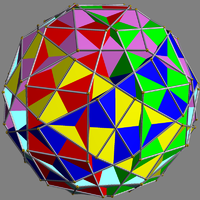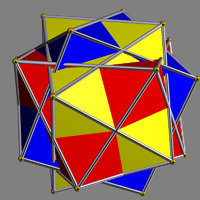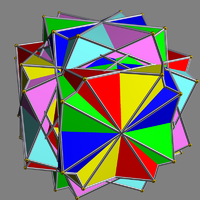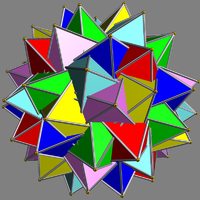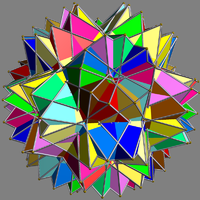• 46-67: Tetrahedral symmetry embedded in octahedral or icosahedral symmetry,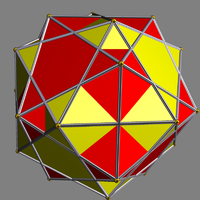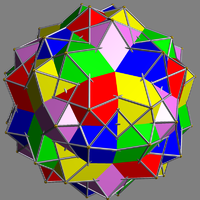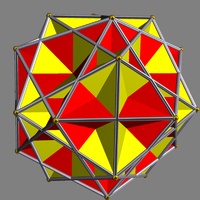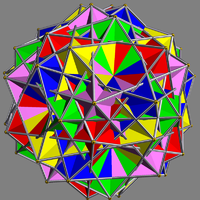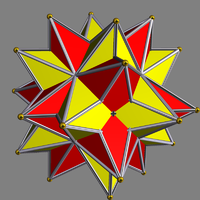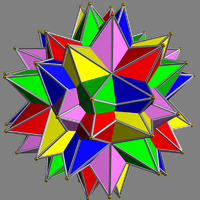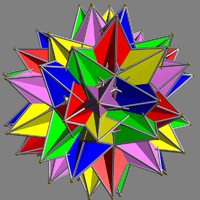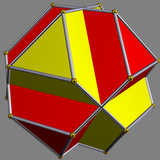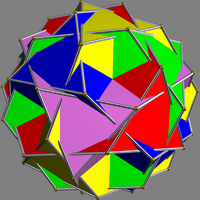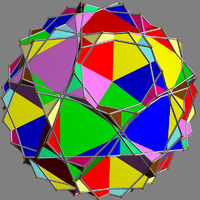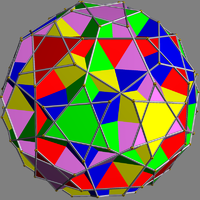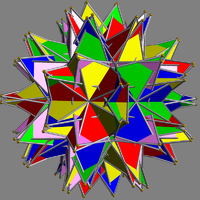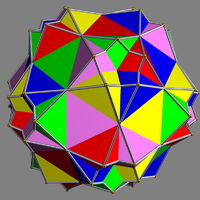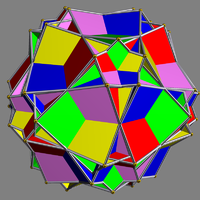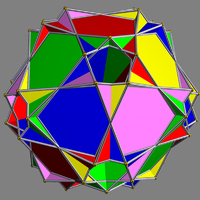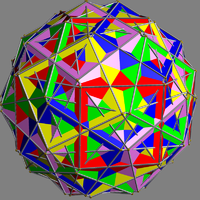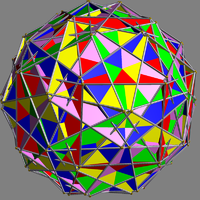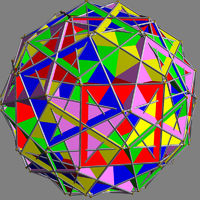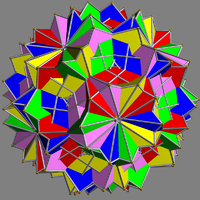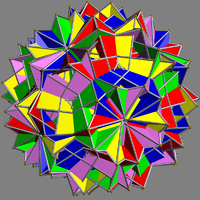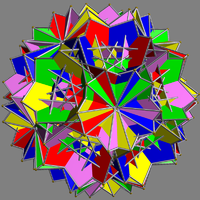• 68-75: enantiomorph pairs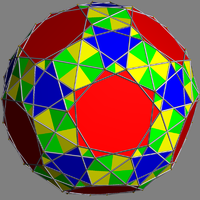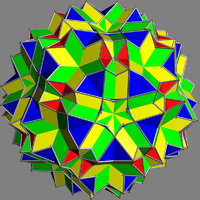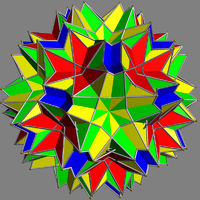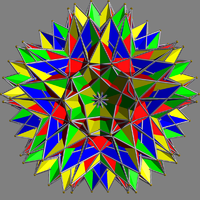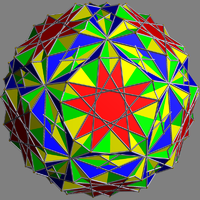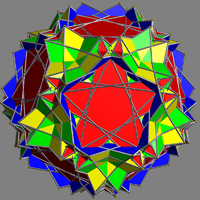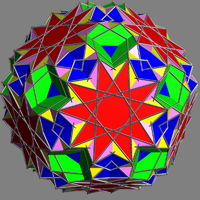Two polyhedra that are compounds but have their elements rigidly locked into place are the small complex icosidodecahedron
Small complex icosidodecahedron
In geometry, the small complex icosidodecahedron is a degenerate uniform star polyhedron. It has 32 faces , 60 edges and 12 vertices...

(compound of icosahedron
Icosahedron
In geometry, an icosahedron is a regular polyhedron with 20 identical equilateral triangular faces, 30 edges and 12 vertices. It is one of the five Platonic solids....

and great dodecahedron) and the great complex icosidodecahedron
Great complex icosidodecahedron
In geometry, the great complex icosidodecahedron is a degenerate uniform star polyhedron. It has 12 vertices, and 60 edges, and 32 faces, 12 pentagrams and 20 pentagons...

(compound of small stellated dodecahedron and great icosahedron). If the definition of a uniform polyhedron
Uniform polyhedron
A uniform polyhedron is a polyhedron which has regular polygons as faces and is vertex-transitive...

is generalised they are uniform.

## Group theory

In terms of group theory
Group theory
In mathematics and abstract algebra, group theory studies the algebraic structures known as groups.The concept of a group is central to abstract algebra: other well-known algebraic structures, such as rings, fields, and vector spaces can all be seen as groups endowed with additional operations and...

, if G is the symmetry group of a polyhedral compound, and the group acts transitively on the polyhedra (so that each polyhedra can be sent to any of the others, as in uniform compounds), then if H is the stabilizer of a single chosen polyhedron, the polyhedra can be identified with the orbit space G/H – the coset gH corresponds to which polyhedron g sends the chosen polyhedron to.